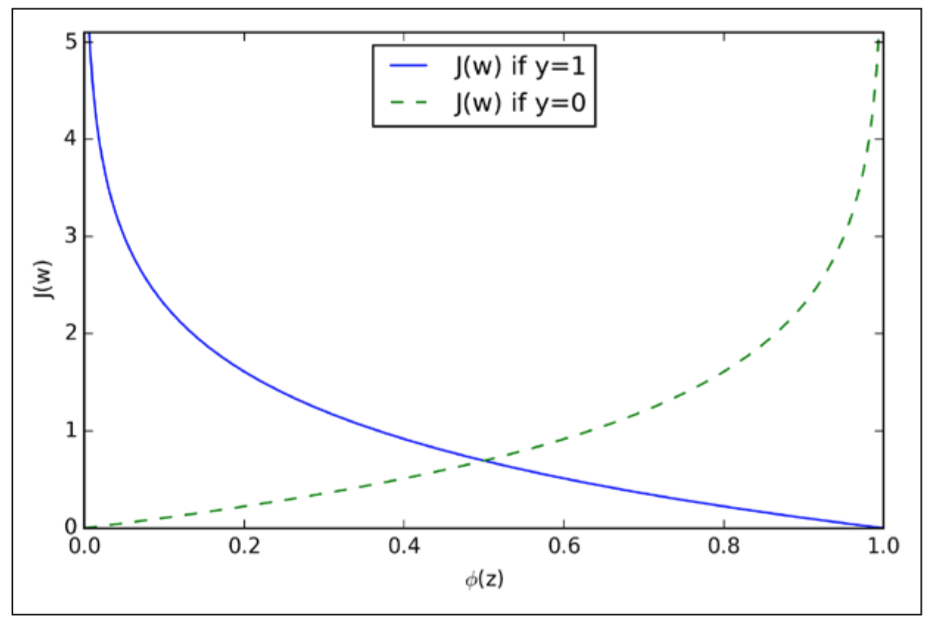# KarlRosaen

Implementing logistic regression by swapping in a new cost function to previous single-layer neural network implementation

Getting back to logistic regression, I again stared at the derivation of the cost function that the Python Machine Learning book provides. It starts with a likelihood function function, a topic I haven't gotten to yet on my All of Statistics book. After reading up a bit on wikipedia, reading ahead in All of Stats, and reviewing the logistic regression section of Advanced Data Analysis from an Elementary Point of View, I decided I get the rough idea and will come back to it later when the stats book gets there.

But suffice it is to say that we can derive a cost function, $J$ that is related to maximizing the log likelihood of the parameters $w$ of our model.

$$J(\mathbf{w}) = \sum_{i=1}^{m} - y^{(i)} log \bigg( \phi\big(z^{(i)}\big) \bigg) - \big(1 - y^{(i)}\big) log\bigg(1-\phi\big(z^{(i)}\big)\bigg).$$

Here $z^{(i)}$ is the linear combination of our weights $w$ and $x^{(i)}$ (the values for each feature in a given sample), $\phi$ is our trusty sigmoid function and $y^{(i)}$ is the correct output. So the cost function penalizes $\phi(z^{(i)})$ being different from $y^{(i)}$:as the book also explains,

We can see that the cost approaches 0 (plain blue line) if we correctly predict that a sample belongs to class 1. Similarly, we can see on the y axis that the cost also approaches 0 if we correctly predict y = 0 (dashed line). However, if the prediction is wrong, the cost goes towards in infinity. The moral is that we penalize wrong predictions with an increasingly larger cost.

With a cost function to apply, the book also notes

If we were to implement logistic regression ourselves, we could simply substitute the cost function J in our Adaline implementation from Chapter 2

The author has published a bonus notebook doing just that, and my goal for today was to implement it as well as part of my chapter 3 work. Mission accomplished!